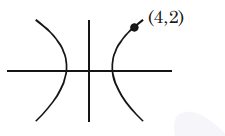# A hyperbola has its centre at the origin,`
Question:

A hyperbola has its centre at the origin, passes through the point $(4,2)$ and has transverse axis of length 4 along the $x$-axis. Then the eccentricity of the hyperbola is:

1. $\frac{2}{\sqrt{3}}$

2. $\frac{3}{2}$

3. $\sqrt{3}$

4. 2

Correct Option: 1

Solution:$\frac{x^{2}}{a^{2}}-\frac{y^{2}}{b^{2}}=1$

$2 a=4 \quad a=2$

$\frac{x^{2}}{4}-\frac{y^{2}}{b^{2}}=1$

Passes through $(4,2)$

$4-\frac{4}{b^{2}}=1 \Rightarrow b^{2}=\frac{4}{3} \Rightarrow e=\frac{2}{\sqrt{3}}$